In this post, I would like to look into Chi-sq goodness of fit test.
During Uni-variate analysis in EDA from the attendance data set, I tried to explain using q-q plot that the feature ‘out-time-diff’ is not normally distributed. To statistically prove the same, we need to use chi square tests.

``````ggplot(data,aes(x = alt.distr)) +
stat_function(fun = dnorm, color="darkred",  size = 1,
args = list(mean = mean(data\$alt.distr),
sd = sd(data\$alt.distr) )) +
geom_density(aes(y=..density..), color="darkblue",  size = 1)+
geom_histogram(aes(y=..density..), bins = 50, fill = "cornflowerblue", alpha = 0.2) +
labs(x = 'Out-time difference (minutes)', y='Density') +
theme_minimal()``````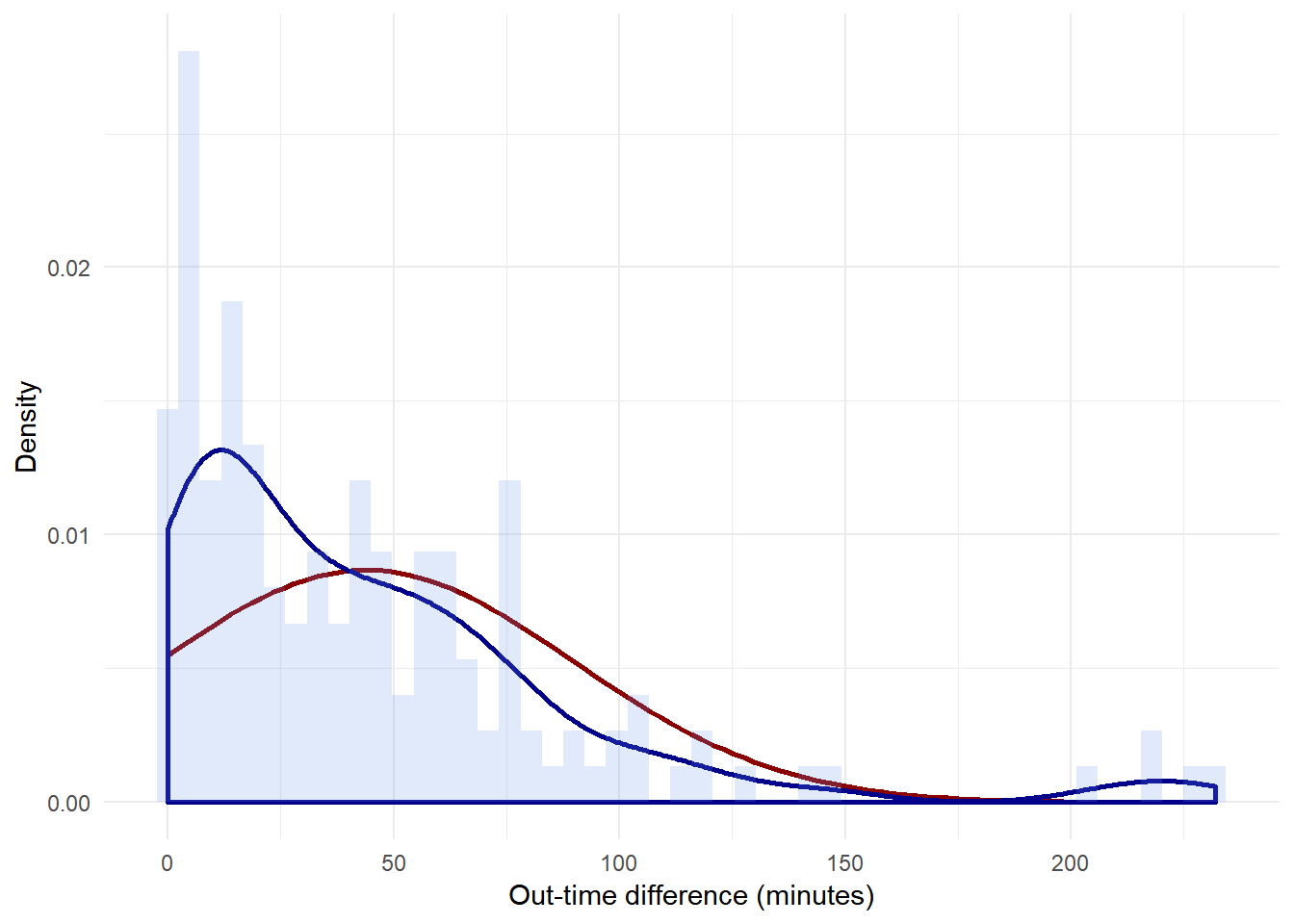The q-q plot for reference:

``````ggplot(data,aes(sample = alt.distr)) +
stat_qq() + stat_qq_line() +
ggtitle("Normal distribution Q-Q plot") +
theme_minimal()``````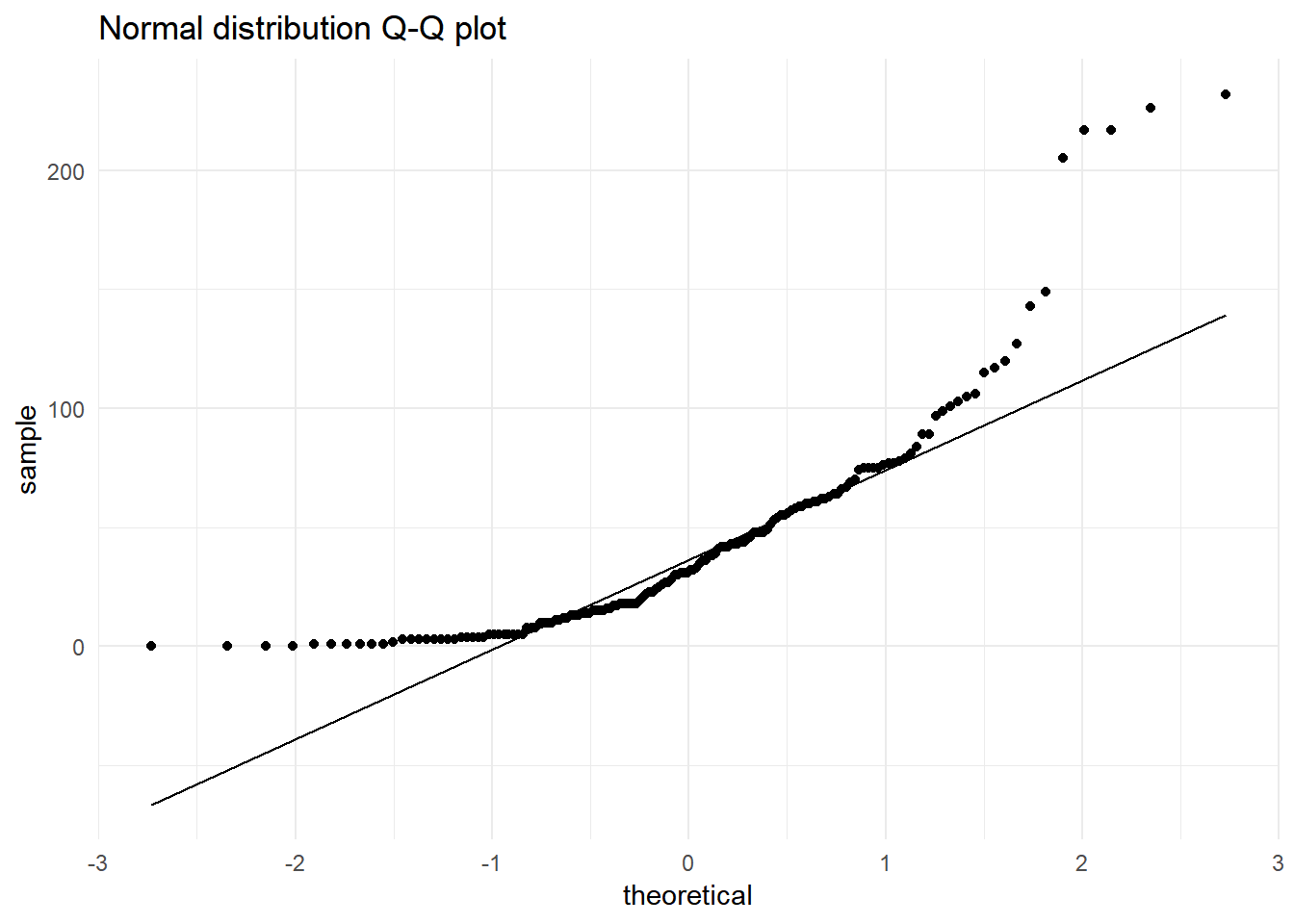The chi-square goodness of fit test for each group would have the following hypothesis:
H0: There is no statistically significant difference between the observed frequencies of ‘diff-in-time’ and expected frequencies from a normal distribution
H1: There is a statistically significant difference

The number of intervals is given by the formula K=1+3.3log(n)

``N <- floor(1+3.3*log10(length(data\$alt.distr)))``

The observed and expected frequencies considering the normal distribution are as follows:

``````minimum.dist <- min(data\$alt.distr)
maximum.dist <- max(data\$alt.distr)
n <- length(data\$alt.distr)
dist.mean <- mean(data\$alt.distr)
dist.sd <- sd(data\$alt.distr)
range.group <- (maximum.dist - minimum.dist)/N
data <- data %>% mutate(class = floor(alt.distr/range.group),
class_name_min =  minimum.dist + class*range.group,
class_name_max = minimum.dist + (class+ 1)*range.group,
class_name = paste0(class_name_min, '-', class_name_max))
chi.sq.table <- data %>% group_by(class, class_name) %>% summarise(obs_freq = n(),
class_name_min = mean(class_name_min),
class_name_max = mean(class_name_max)) %>%
mutate(exp_freq = pnorm(class_name_max, dist.mean, dist.sd)*n - pnorm(class_name_min, dist.mean, dist.sd)*n,
chi.sq = ((obs_freq - exp_freq)^2)/exp_freq)``````
``````library(kableExtra)
kable(chi.sq.table %>% dplyr::select(class_name, obs_freq, exp_freq, chi.sq),
caption = 'Contingency Table') %>%
kable_styling(full_width = F) %>%
column_spec(1, bold = T) %>%
collapse_rows(columns = 1:2, valign = "middle") %>%
scroll_box()``````
Contingency Table
class class_name obs_freq exp_freq chi.sq
0 0-29 74 32.0974138 54.7030591
1 29-58 37 39.1354970 0.1165271
2 58-87 28 32.4557899 0.6117264
3 87-116 9 18.3059346 4.7307292
4 116-145 4 7.0201144 1.2992795
5 145-174 1 1.8295669 0.3761443
7 203-232 4 0.0389060 403.2865367
8 232-261 1 0.0031698 313.4780888

Performing the Chi-square goodness of fit test:

``````# Functions used in chi-sq-test

chi.sq.plot <- function(pop.mean=0, alpha = 0.05, chi.sq, df,
label = 'Chi Square distribution',title = 'Chi Square goodness of fit test'){
# Creating a sample chi-sq distribution
range <- seq(qchisq(0.0001, df), qchisq(0.9999, df), by = (qchisq(0.9999, df)-qchisq(0.0001, df))*0.001)
chi.sq.dist <- data.frame(range = range, dist = dchisq(x = range, ncp = pop.mean, df = df)) %>%
dplyr::mutate(H0 = if_else(range <= qchisq(p = 1-alpha, ncp = pop.mean, df = df,lower.tail = TRUE),'Retain', 'Reject'))
# Plotting sampling distribution and x_bar value with cutoff
plot.test <- ggplot(data = chi.sq.dist, aes(x = range,y = dist)) +
geom_area(aes(fill = H0)) +
scale_color_manual(drop = TRUE, values = c('Retain' = "#00BFC4", 'Reject' = "#F8766D"), aesthetics = 'fill') +
geom_vline(xintercept = chi.sq, size = 2) +
geom_text(aes(x = chi.sq, label = paste0('Chi Sq = ', round(chi.sq,3)), y = mean(dist)), colour="blue", vjust = 1.2) +
labs(x = label, y='Density',  title = title) +
theme_minimal()+theme(legend.position="bottom")
plot(plot.test)
}``````
``````chi.test <- chisq.test(chi.sq.table\$obs_freq, p = chi.sq.table\$exp_freq, rescale.p = TRUE)
print(chi.test)``````
``````##
##  Chi-squared test for given probabilities
##
## data:  chi.sq.table\$obs_freq
## X-squared = 640.34, df = 7, p-value < 2.2e-16``````
``chi.sq.plot(chi.sq = chi.test\$statistic, df = chi.test\$parameter, title = 'Null hypothesis to test normality')``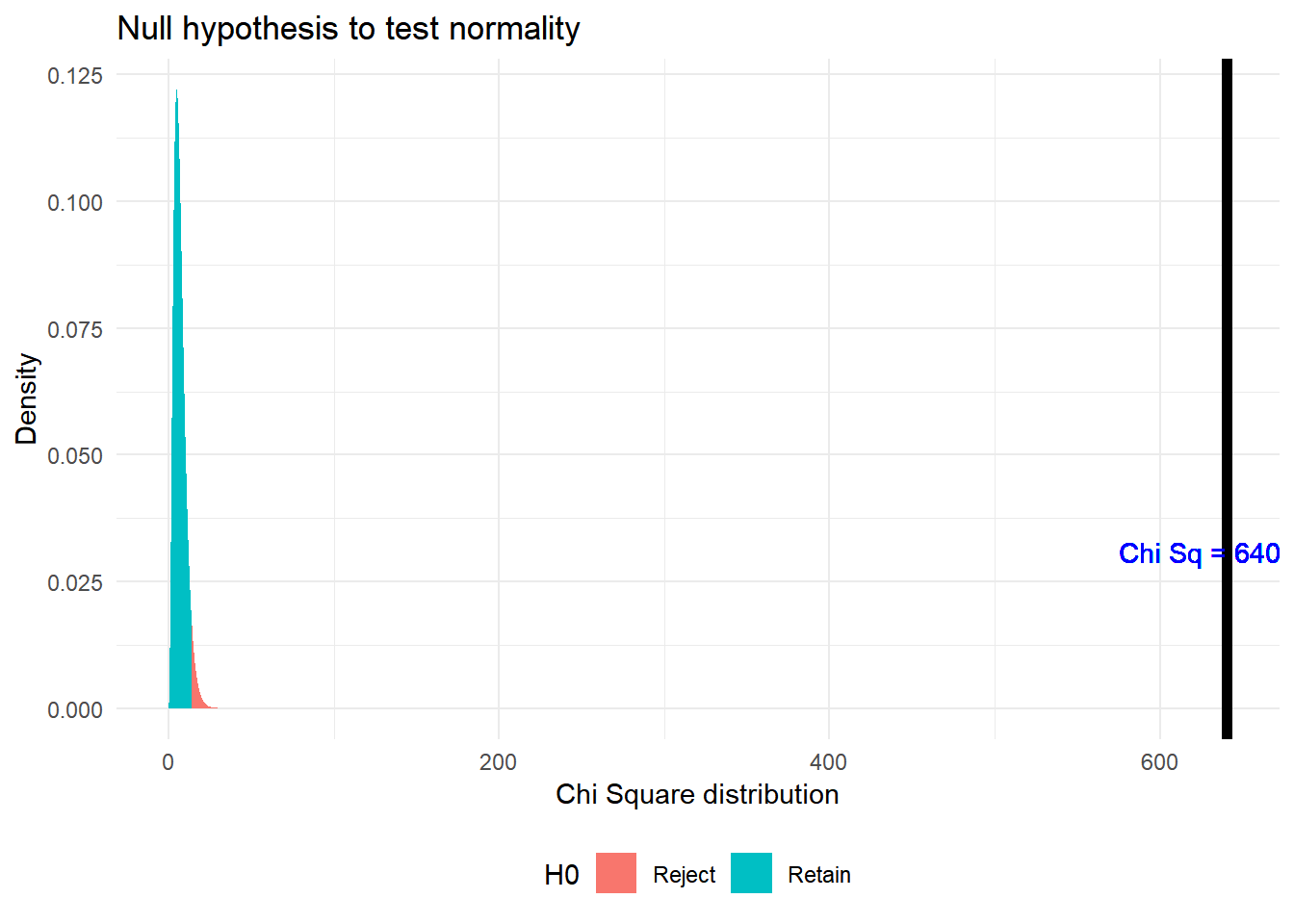As p < α, where α = 0.05, rejecting the Null hypothesis. therefore the distribution is not a normal distribution.

In the same post, I showed using qq plot how the distribution looks like a exponential distribution. To test if it is statistically significant, I can use the same goodness of fit test.
The chi-square goodness of fit test for each group would have the following hypothesis:
H0: There is no statistically significant difference between the observed frequencies of ‘diff-in-time’ and expected frequencies from exponential distribution
H1: There is a statistically significant difference

``````minimum.dist <- min(data\$alt.distr)
maximum.dist <- max(data\$alt.distr)
n <- length(data\$alt.distr)
lamda <- 1/mean(sd(data\$alt.distr),mean(data\$alt.distr))
range.group <- (maximum.dist - minimum.dist)/N
data <- data %>% mutate(class = floor(alt.distr/range.group),
class_name_min =  minimum.dist + class*range.group,
class_name_max = minimum.dist + (class+ 1)*range.group,
class_name = paste0(class_name_min, '-', class_name_max))
chi.sq.table <- data %>% group_by(class, class_name) %>% summarise(obs_freq = n(),
class_name_min = mean(class_name_min),
class_name_max = mean(class_name_max)) %>%
mutate(exp_freq = pexp(class_name_max, lamda)*n - pexp(class_name_min, lamda)*n,
chi.sq = ((obs_freq - exp_freq)^2)/exp_freq)``````
Contingency Table
class class_name obs_freq exp_freq chi.sq
0 0-29 74 73.9534204 0.0000293
1 29-58 37 39.3388103 0.1390493
2 58-87 28 20.9259016 2.3914319
3 87-116 9 11.1313320 0.4080892
4 116-145 4 5.9212050 0.6233577
5 145-174 1 3.1497280 1.4672157
7 203-232 4 0.8912488 10.8435869
8 232-261 1 0.4740912 0.5833899

I observe that the expected frequency value is close to the observed frequency value in most of the cases.

``````##
##  Chi-squared test for given probabilities
##
## data:  chi.sq.table\$obs_freq
## X-squared = 16.194, df = 7, p-value = 0.0234``````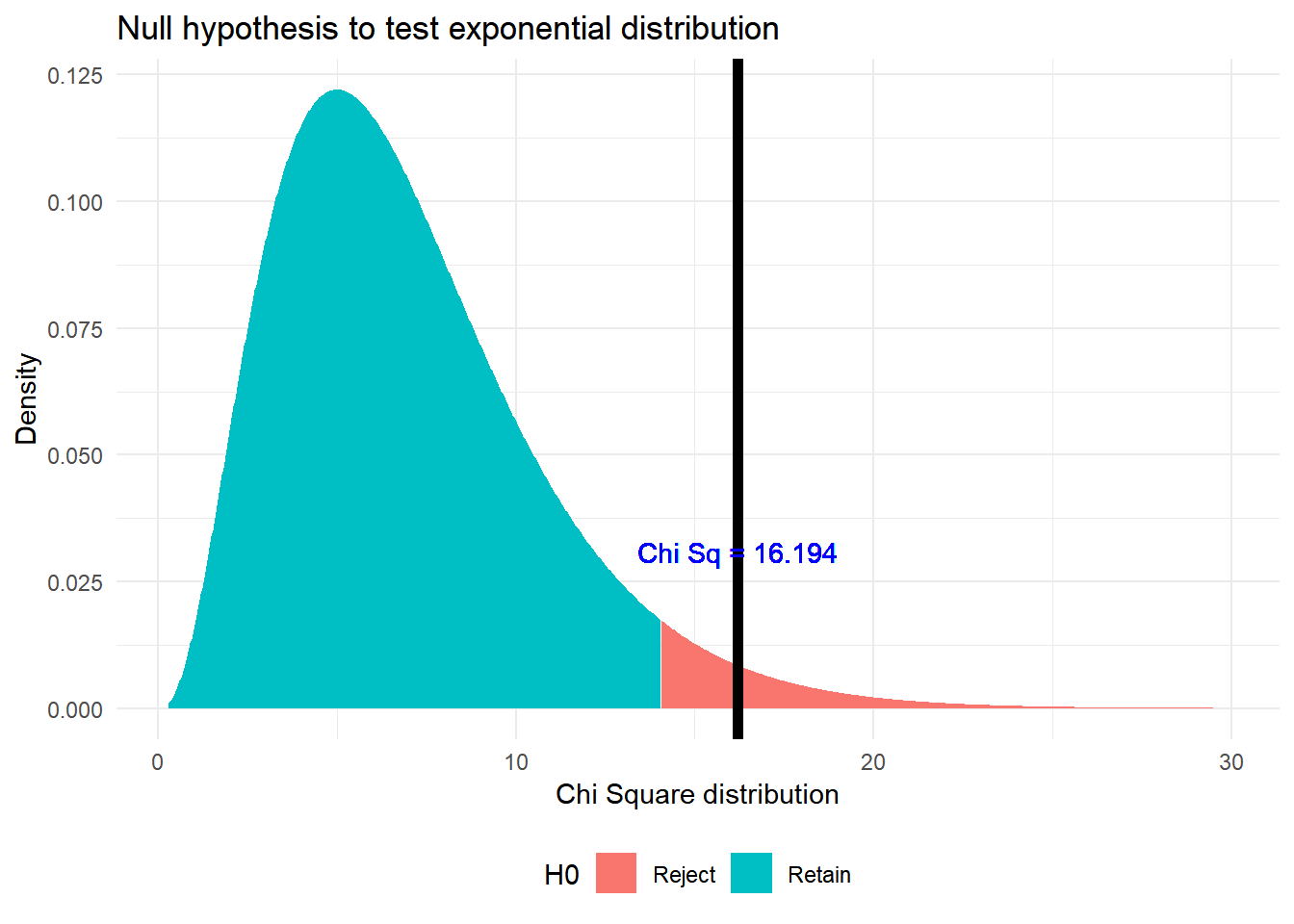From the table above, I can see that most of the high chi-sq values are due to Class 7 where observed frequency is less than 10. Ignoring outlier cases with less than 10 frequency, I get:

``````##
##  Chi-squared test for given probabilities
##
## data:  chi.sq.table\$obs_freq
## X-squared = 2.8385, df = 3, p-value = 0.4172``````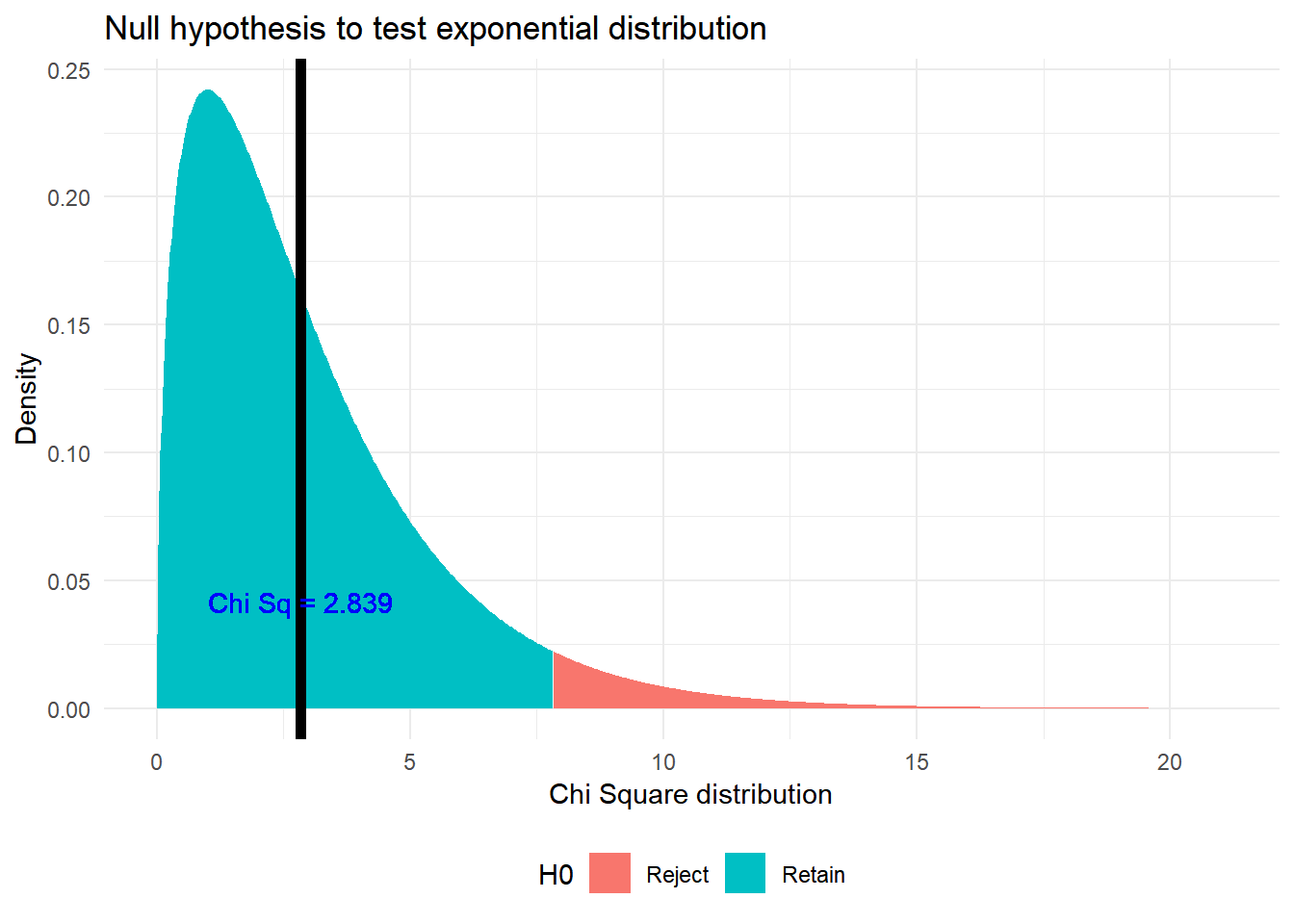As p > α, where α = 0.05, retaining the Null hypothesis
I want to find the effect size or the strength of relationship between these variables. That is explained by Cramers V by``````library(lsr)
cramersV(chi.sq.table\$obs_freq, p = chi.sq.table\$exp_freq, rescale.p = TRUE)``````
``##  0.03988245``

For df=3 from Cramers table I get {small effect = .06, medium effect = .17, large effect = .29} The current effect is very small.

Created on 23rd June 2019, Achyuthuni Sri Harsha